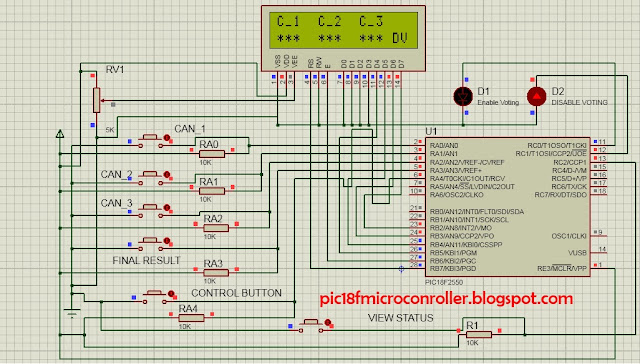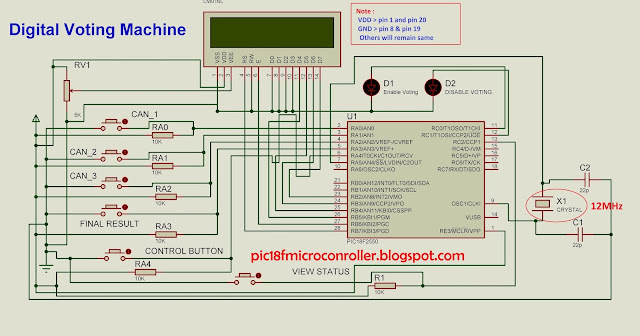# Learn Embedded Programming [Step by Step]

## Digital Voting Machine Project Using PIC Microcontroller

Digital Voting Machine  is one kind of microcontroller based device which can be performed in election . Once I had made Digital Voting Machine when I was in 6th semester. Today I am going to share an upgrade version of  it . In the upgrade version I used  EEPROM of  PIC 18f2550  so that data can be saved during electricity failure. Now take a look on the picture :Digital Voting Machine Circuit

### Description :

This Voting Machine conains 6 buttons for operation and two LEDs  for notification.

#### Candidate Category Button :

Button_RA0 : Candidate_1 .

Button_RA1 : Candidate_2 .

Button_RA2 : Candidate_3 .

#### Controling Button :

Button_RA4 : Control Button.

#### Status View Button :

Button_RC2 :  Status View  Button.

#### Result Button :

Button_RA3 : Final Result Button.

### ***Controling Button :

This is the main controller of all other buttons. Without having permission of  this button, any button can't perform. Without permission, reading instruction will not be taken. This will be placed in the presiding officer's room. Once he press the button and only one of these buttons can be permitted to perform only for one time. If any key or button is pressed, it works for one time and the system becomes disable automatically for voting until the presiding officer press control button again. So, one person will be able to vote for one time & voter will not be able to do that without permission of PO. Watch this video to understand completely.
Video of  Digital Voting Machine in Proteus :digital voting machine

### ***Status View Button :

For security reason we are hiding the voting status from the voter and Only the PO(presiding officer) can check this. At first PO have to press "Control Button " and  LED 1 turns on when he can see the voting status by pressing "View Status" button. Current status will be shown only for a little time before getting back to the previous stage with turning on LED2.

### *** Result Button :

When OP decide to publish who is winner , he just need to press "control button" and after that need to press Final Result Button . After Showing result , it will be reset and all data will be erased .

### ***Candidate Category Button :

When control button gives permission , only one of  "candidate button category" buttons can perform,  only for one time . After performing,  this will disable the system . Generally these buttons are incremented by one during each action .

***LED 1 & LED 2 :

When LED1 remains on , others buttons can perform . When LED2 remains on, all buttons are disabled.

### Source Code :

``````

sbit LCD_RS at RB7_bit;
sbit LCD_EN at RB6_bit;
sbit LCD_D4 at RB5_bit;
sbit LCD_D5 at RB4_bit;
sbit LCD_D6 at RB3_bit;
sbit LCD_D7 at RB2_bit;
sbit LCD_RS_Direction at TRISB7_bit;
sbit LCD_EN_Direction at TRISB6_bit;
sbit LCD_D4_Direction at TRISB5_bit;
sbit LCD_D5_Direction at TRISB4_bit;
sbit LCD_D6_Direction at TRISB3_bit;
sbit LCD_D7_Direction at TRISB2_bit;
// End LCD module connections
short dat_can1=0,dat_can2=0,dat_can3=0,con=4;
char txt[]="        ";
char txt1[]="htp://pic18fmicrocontroller.blogspot.com";
char txt2[]="    Digital Voting Machine";
int i=0,j=0,chk=10;
char c1i='0',c1j='0',c1k='0';
char c2i,c2j,c2k;
char c3i,c3j,c3k;
int chkk = 33,aq=0,b=0,aa=0,bb=0,cc=0;
char thirdchar(short dk){         ////find third Char of Short Data
aq=dk/100;
aa=aq*100;
aa=dk-aa;
if(aq==0)
{ aa=dk;
return '0'; }
if(aq==1) return '1';
if(aq==2) return '2';
if(aq==3) return '3';
if(aq==4) return '4';
if(aq==5) return '5';
if(aq==6) return '6';
if(aq==7) return '7';
if(aq==8) return '8';
if(aq==9) return '9';
}
char secondchar(short dk){  ////find Second Char of Short Data
b=aa/10;
bb=b*10;
bb=aa-bb;
if(b==0)
{ bb=dk;
return '0'; }
if(b==1) return '1';
if(b==2) return '2';
if(b==3) return '3';
if(b==4) return '4';
if(b==5) return '5';
if(b==6) return '6';
if(b==7) return '7';
if(b==8) return '8';
if(b==9) return '9';
}
char firstchar(short dk){ ////find first Char of Short Data
if(bb==0) return '0';
if(bb==1) return '1';
if(bb==2) return '2';
if(bb==3) return '3';
if(bb==4) return '4';
if(bb==5) return '5';
if(bb==6) return '6';
if(bb==7) return '7';
if(bb==8) return '8';
if(bb==9) return '9';
}
void main() {
CMCON=7;
TRISA.F0=1;
TRISA.F1=1;
TRISA.F2=1;
TRISA.F3=1;
TRISA.F4=1;
TRISC.F0=0;
TRISC.F1=0;
TRISC.F2=1;
Lcd_Init();
Lcd_Cmd(_LCD_CLEAR);        // Clear display
Lcd_Cmd(_LCD_CURSOR_OFF);
/////////////////////// Lcd Scroling Display Start
for(i=0;i<19;i++){
Lcd_Out(1,1,txt1);
Lcd_Out(2,1,txt2);
Lcd_Cmd(_LCD_SHIFT_LEFT);
delay_ms(200);
}
Lcd_Cmd(_LCD_CLEAR);
/////////////////////// Lcd Scroling Display End
for(j=0;j<16;j++){
Lcd_Cmd(_LCD_CLEAR);
txt[j]='.';
Lcd_Out(1,1," Starting...");
Lcd_Out(2,1,txt);
delay_ms(300);
}
c1i=thirdchar(dat_can1); ///third
c1j=secondchar(dat_can1); //second
c1k=firstchar(dat_can1);   //first
//// these functions should be called with this structure
/// I used this , because MikroC's short to str conversion didn't work .
c2i=thirdchar(dat_can2);
c2j=secondchar(dat_can1);
c2k=firstchar(dat_can1);
c3i=thirdchar(dat_can3);
c3j=secondchar(dat_can1);
c3k=firstchar(dat_can1);
Lcd_Cmd(_LCD_CLEAR);
if(dat_can3<=0){    // check if it runs for the first time , then set data null or 0
}
if(dat_can2<=0){      // check if it runs for the first time , then set data null or 0
}
if(dat_can1<=0){       // check if it runs for the first time , then set data null or 0
}
while(1){
c1i=thirdchar(dat_can1);   // It is taking the last update data for Candidate 1
c1j=secondchar(dat_can1);
c1k=firstchar(dat_can1);
c2i=thirdchar(dat_can2);    // It is taking the last update data for Candidate 2
c2j=secondchar(dat_can2);
c2k=firstchar(dat_can2);
c3i=thirdchar(dat_can3);        // It is taking the last update data for Candidate 3
c3j=secondchar(dat_can3);
c3k=firstchar(dat_can3);
Lcd_Out(1,1,"C_1 C_2 C_3");
Lcd_Chr(2,1,'*');
Lcd_Chr(2,2,'*');
Lcd_Chr(2,3,'*');
Lcd_Chr(2,6,'*');
Lcd_Chr(2,7,'*');
Lcd_Chr(2,8,'*');
Lcd_Chr(2,11,'*');
Lcd_Chr(2,12,'*');
Lcd_Chr(2,13,'*');
if(PORTA.F4==0){  // if control button is pressed , it enables voting.
con=3;
}
if(con!=3){    // if control button is not pressed ,naturally it disables voting.
Lcd_Out(2,15,"DV");
PORTC.F0=0;
PORTC.F1=1;     //LED2 is on
}
while(con==3){    // if control button is pressed , it enables voting.
Lcd_Out(2,15,"EV");
PORTC.F0=1;     //LED1 is on
PORTC.F1=0;
if(PORTC.F2==0){   // when view status button is pressed
Lcd_Chr(2,1,c1i);
Lcd_Chr(2,2,c1j);
Lcd_Chr(2,3,c1k);
Lcd_Chr(2,6,c2i);
Lcd_Chr(2,7,c2j);
Lcd_Chr(2,8,c2k);
Lcd_Chr(2,11,c3i);
Lcd_Chr(2,12,c3j);
Lcd_Chr(2,13,c3k);
delay_ms(4000);
con=5;    // con=5 makes disable voting
}
if(PORTA.F0==0){
dat_can1=dat_can1+1;   // Candidate 1 variable is incrementing .
EEPROM_Write(can_1adrs,dat_can1); // writing the incremented value on EEPROM for Candidate 1
con=5;    // con=5 makes disable voting
}
if(PORTA.F1==0){
dat_can2=dat_can2+1;      // Candidate 2 variable is incrementing .
EEPROM_Write(can_2adrs,dat_can2);   // writing the incremented value on EEPROM for Candidate 2
con=5;                  // con=5 makes disable voting
}
if(PORTA.F2==0){
dat_can3=dat_can3+1;          // Candidate 3 variable is incrementing .
EEPROM_Write(can_3adrs,dat_can3);     // writing the incremented value on EEPROM for Candidate 3
con=5;                   // con=5 makes disable voting
}
if(PORTA.F3==0){      // If Result Button is pressed .
Lcd_Cmd(_LCD_CLEAR);
for(j=1;j<17;j++){
Lcd_Out(1,1," Calculating...");
Lcd_Out(2,j,".");
delay_ms(200);
}
if(dat_can2>dat_can1&&dat_can2>dat_can3){
Lcd_Cmd(_LCD_CLEAR);
Lcd_Out(1,1,"Winner is C_2");
Lcd_Out(2,1,"Congratulation!!");
delay_ms(5000);
Lcd_Out(1,1,"Winner is C_2");
Lcd_Out(2,1,"He got =");
delay_ms(5000);
Lcd_Cmd(_LCD_CLEAR);
dat_can2=0;
//Erasing All Datas
dat_can1=0;
dat_can3=0;
}
else if(dat_can1>dat_can2&&dat_can1>dat_can3){
Lcd_Cmd(_LCD_CLEAR);
Lcd_Out(1,1,"Winner is C_1");
Lcd_Out(2,1,"Congratulation!!");
delay_ms(5000);
Lcd_Cmd(_LCD_CLEAR);
dat_can2=0;
dat_can1=0;
dat_can3=0;
}
else if(dat_can3>dat_can1&&dat_can3>dat_can2){
Lcd_Cmd(_LCD_CLEAR);
Lcd_Out(1,1,"Winner is C_3");
Lcd_Out(2,1,"Congratulation!!");
delay_ms(5000);
Lcd_Cmd(_LCD_CLEAR);
dat_can2=0;
dat_can1=0;
dat_can3=0;
}
else{
Lcd_Cmd(_LCD_CLEAR);
Lcd_Out(1,1,"Something is");
Lcd_Out(2,1,"Worng!!!");
delay_ms(1000);
Lcd_Cmd(_LCD_CLEAR);
}
con=5;
}
}
}
}
``````

### Circuit Diagram :Digital Voting Machine Using Microcontroller

Video of  Digital Voting Machine in Practical :

## Thank You.

Ain't getting any visitors!

## Featured Post

### Bluetooth Based Temperature Meter Project using Microcontroller and MikroC USART Terminal## Tags

: (1) 18F2550 (1) 36KHz (3) and (1) arduino (1) Based (1) battery (1) Bipolar (1) Blinking (1) blinks (1) Bluetooth (1) button (1) circuit (1) clock (1) control (1) Db9 (1) DC Motor (2) digital (2) display (2) DS1307 (1) electronic (1) flash (1) flashing (1) HC-06 (1) home (1) how (1) How to (10) i2c tutorial (1) in (1) indicator (1) interface (8) interfacing (3) Interrupt (3) Introduction (1) IR Receiver (4) key pad (1) keyboard (1) keypad (1) lavel (1) Lcd 16x2 (2) lcd 2x16 (2) led (1) lm35 (2) LPG (1) machine (1) make (1) making (1) matrix (1) max232 (1) meter (2) microchip (4) microchips (3) mikroC (5) musical (1) NEC Protocol (4) pcb (5) PIC (3) pic controller (11) pic proteus (1) Pic Tutorial (12) pic18 (2) pic18f2550 (11) picRFモジュール (1) proteus (6) push (1) push button (1) PWM (1) real (1) Rs 232 (1) Rs232 (1) scroll (1) scrolling (1) Serial Port (1) simulation (2) step by step (7) step bystep (1) text (2) time (1) timer (4) timer0 (4) tone (1) tutorial (2) Unipolar (1) USB (1) usb 1.0 (1) USB HID (1) using (9) voltmeter (1) voting (1) with (2) work (1)

## Live Traffic Feed

Live Traffic Feed
Visitor Tracking